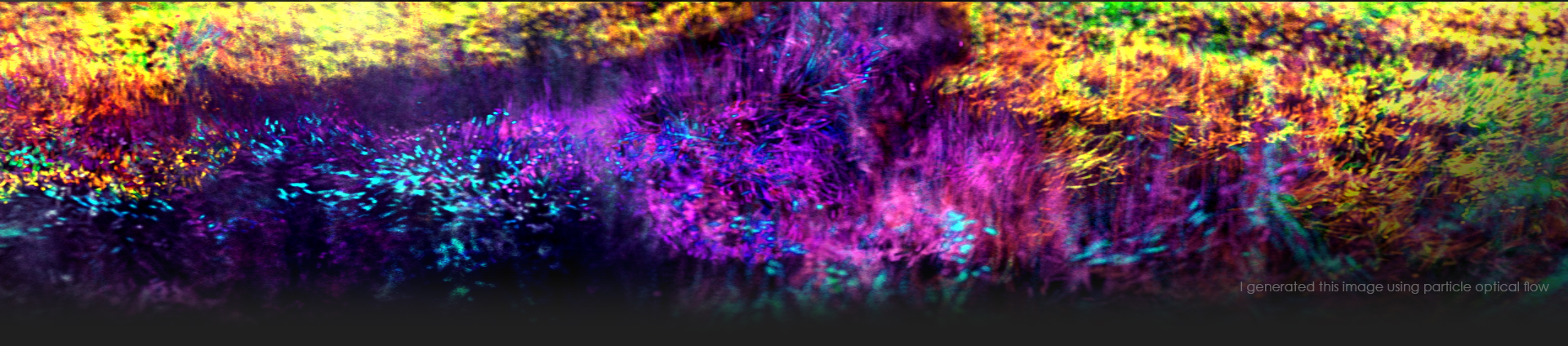474

18 Good

• Rank
Illusionist

• Name
Masoud
• Location
Iran

Recent Profile Visitors

2,746 profile views

• JXS

1. Hi Atom, I want to use my custom collision object, so why a "groundplane" works fine, but my collision doesn't?
2. Hmmm, OK, thanks.
3. Hello I would like to create a Fluid-RBD interaction. But as you can see in the image below, the fluid pushes the rigid body so strongly. I need the rigid body to be a little bit heavy and to float on the fluid, based on its mass attribute. (Decreasing the "Feedback Scale", didn't help). Thanks for helping. RBD-FLIP Interaction_01.hip
4. Hi guys, How can I make an interaction between a FLIP-Fluid and a vellum object? Is it possible? Thanks for helping. FLIP and Vellum Interaction_01.hip
5. Hello guys, In a simple RBD simulation, when I replace the "Ground Plane" collision DOP with my Collision-Box, the rigid body objects never stop moving! How can I bring them to stop? (When I use the "Ground Plane" node, everything is ok!). I tried to use the " rbdautofreeze DOP", but it doesn't work. Thanks for helping. RBDs_01.hip
6. Hi Tesan, Than you. Yes, it works fine: vector4 q1 = quaternion(@N); vector4 q2 = dihedral(v@N, v@targetVector); vector4 quat = slerp( q1, q2, chf("bias")); v@N = qrotate(quat, v@N);
7. Hi Konstantin; Thank you for the reply. Actually, I want to rotate scattered points' Normal to another direction (i.e. MyVector) using a blend amount. At first, I tried the lerp() function but instead of rotation, it blends them. Then I tried: float angle = chf("Angle"); vector4 rot = quaternion(radians(angle), v@myVector); v@N = qrotate(rot, @N); But it rotates the Normal around "My-Vector"! Here is an image of what I need: Rotate_Vector.hip
8. Hello guys, Using VEX, how can I rotate a vector to another vector, by a blend value from zero to one (i.e 1=fully aligned, 0= not-aligned)? Thanks for helping.
9. Hello, In Houdini 19, the "Resample SOP" isn't work as before when I set the "Treat Polygon As" to "Subdivision Curves"..! Is this a bug? Thanks for helping. Resample Curve.hip
10. Hello guys, As you can see in the image below, in a bunch of points islands, I need these things, using VEX: 1) I want to apply random color to each point island (cluster). 2) I want to group each cluster’s (averaged) center points. I tried several ways without success . I would appreciate any ideas. Thanks for helping. Center_Of_Point_Clusters.hip
11. Any idea?
12. Hello guys, As you know, the default attribute value of "Density" in Flip-Fluid is 1000. Now, in a simulation with two amounts of "Densities" (e.g 1000 and 100), what is the best way to speed up the rising speed of low-density fluid particles? I want to force them to reach the fluid surface as fast as possible (maybe something like the buoyancy parameter, in the pyro solver). Thanks for helping.
×